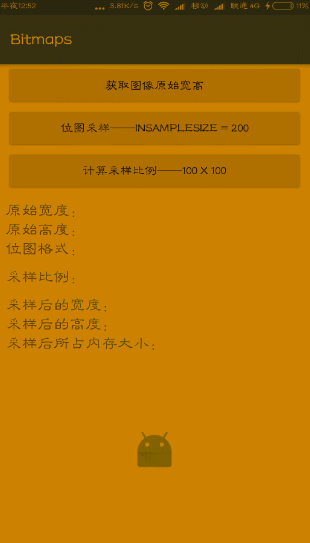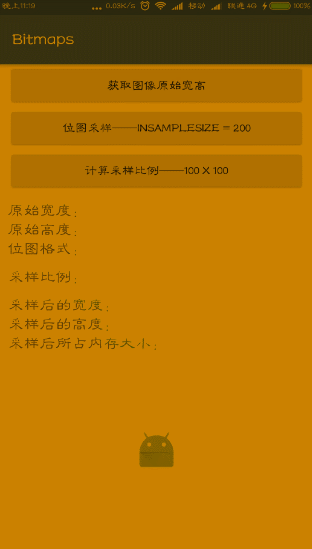Bitmap之位图采样和内存计算详解

Android 开发中经常考虑的一个问题就是 OOM(Out Of Memory)，也就是内存溢出，一方面大量加载图片时有可能出现 OOM， 通过采样压缩图片可避免 OOM，另一方面，如一张 1024 x 768 像素的图像被缩略显示在 128 x 96 的 ImageView 中，这种做法显然是不值得的，可通过采样加载一个合适的缩小版本到内存中，以减小内存的消耗，Bitmap 的优化主要有两个方面如下，一是有效的处理较大的位图，二是位图的缓存，其中位图缓存对应文章如下：

1. 估计加载完整图像所需要的内存
2. 加载这个图片所需的空间带给其程序的其他内存需求
3. 加载图片的目标 ImageView 或 UI 组件的尺寸
4. 当前设备的屏幕尺寸或密度

位图采样

/**
* 位图采样
* @param res
* @param resId
* @return
*/
public Bitmap decodeSampleFromResource(Resources res, int resId){
//BitmapFactory创建设置选项
BitmapFactory.Options options = new BitmapFactory.Options();
//设置采样比例
options.inSampleSize = 200;
Bitmap bitmap = BitmapFactory.decodeResource(res,resId,options);
return bitmap;
}

/**
* 1.计算位图采样比例
*
* @param option
* @param reqWidth
* @param reqHeight
* @return
*/
public int calculateSampleSize(BitmapFactory.Options option, int reqWidth, int reqHeight) {
//获得图片的原宽高
int width = option.outWidth;
int height = option.outHeight;

int inSampleSize = 1;
if (width > reqWidth || height > reqHeight) {
if (width > height) {
inSampleSize = Math.round((float) height / (float) reqHeight);
} else {
inSampleSize = Math.round((float) width / (float) reqWidth);
}
}
return inSampleSize;
}

/**
* 2.计算位图采样比例
* @param options
* @param reqWidth
* @param reqHeight
* @return
*/
public int calculateSampleSize1(BitmapFactory.Options options, int reqWidth, int reqHeight) {

//获得图片的原宽高
int height = options.outHeight;
int width = options.outWidth;

int inSampleSize = 1;
if (height > reqHeight || width > reqWidth) {
// 计算出实际宽高和目标宽高的比率
final int heightRatio = Math.round((float) height / (float) reqHeight);
final int widthRatio = Math.round((float) width / (float) reqWidth);
/**
* 选择宽和高中最小的比率作为inSampleSize的值，这样可以保证最终图片的宽和高
* 一定都会大于等于目标的宽和高。
*/
inSampleSize = heightRatio < widthRatio ? heightRatio : widthRatio;
}
return inSampleSize;
}

/**
* 位图采样
* @param resources
* @param resId
* @param reqWidth
* @param reqHeight
* @return
*/
public Bitmap decodeSampleFromBitmap(Resources resources, int resId, int reqWidth, int reqHeight) {
//创建一个位图工厂的设置选项
BitmapFactory.Options options = new BitmapFactory.Options();
//设置该属性为true,解码时只能获取width、height、mimeType
options.inJustDecodeBounds = true;
//解码
BitmapFactory.decodeResource(resources, resId, options);
//计算采样比例
int inSampleSize = options.inSampleSize = calculateSampleSize(options, reqWidth, reqHeight);
//设置该属性为false，实现真正解码
options.inJustDecodeBounds = false;
//解码
Bitmap bitmap = BitmapFactory.decodeResource(resources, resId, options);
return bitmap;
}

/**
* 解码指定id的资源文件
*/
public static Bitmap decodeResource(Resources res, int id, BitmapFactory.Options opts) {
...
/**
* 根据指定的id打开数据流读取资源，同时为TypeValue进行复制获取原始资源的density等信息
* 如果图片在drawable-xxhdpi，那么density为480dpi
*/
is = res.openRawResource(id, value);
//从输入流解码出一个Bitmap对象，以便根据opts缩放相应的位图
bm = decodeResourceStream(res, value, is, null, opts);
...
}

/**
* 从输入流中解码出一个Bitmap，并对该Bitmap进行相应的缩放
*/
public static Bitmap decodeResourceStream(Resources res, TypedValue value,
InputStream is, Rect pad, BitmapFactory.Options opts) {

if (opts == null) {
//创建一个默认的Option对象
opts = new BitmapFactory.Options();
}

/**
* 如果设置了inDensity的值，则按照设置的inDensity来计算
* 否则将资源文件夹所表示的density设置inDensity
*/
if (opts.inDensity == 0 && value != null) {
final int density = value.density;
if (density == TypedValue.DENSITY_DEFAULT) {
opts.inDensity = DisplayMetrics.DENSITY_DEFAULT;
} else if (density != TypedValue.DENSITY_NONE) {
opts.inDensity = density;
}
}

/**
* 同理，也可以通过BitmapFactory.Option对象设置inTargetDensity
* inTargetDensity 表示densityDpi，也就是手机的density
* 使用DisplayMetrics对象.densityDpi获得
*/
if (opts.inTargetDensity == 0 && res != null) {
opts.inTargetDensity = res.getDisplayMetrics().densityDpi;
}
//decodeStream()方法中调用了native方法
}

/**
* 返回解码后的Bitmap,
*/
public static Bitmap decodeStream(InputStream is, Rect outPadding, BitmapFactory.Options opts) {
...
Set the newly decoded bitmap's density based on the Options
//根据Options设置最新解码的Bitmap
setDensityFromOptions(bm, opts);
...
return bm;
}

/**
* BitmapFactory.cpp 源码
*/
static jobject doDecode(JNIEnv*env, SkStreamRewindable*stream, jobject padding, jobject options) {
...
if (env -> GetBooleanField(options, gOptions_scaledFieldID)) {
const int density = env -> GetIntField(options, gOptions_densityFieldID);
const int targetDensity = env -> GetIntField(options, gOptions_targetDensityFieldID);
const int screenDensity = env -> GetIntField(options, gOptions_screenDensityFieldID);
if (density != 0 && targetDensity != 0 && density != screenDensity) {
//计算缩放比例
scale = (float) targetDensity / density;
}
}
...
//原始Bitmap
SkBitmap decodingBitmap;
...

//原始位图的宽高
int scaledWidth = decodingBitmap.width();
int scaledHeight = decodingBitmap.height();

//综合density和targetDensity计算最终宽高
if (willScale && decodeMode != SkImageDecoder::kDecodeBounds_Mode) {
scaledWidth = int(scaledWidth * scale + 0.5f);
scaledHeight = int(scaledHeight * scale + 0.5f);
}
...
//x、y方向上的缩放比例，大概与scale相等
const float sx = scaledWidth / float(decodingBitmap.width());
const float sy = scaledHeight / float(decodingBitmap.height());
...
//将canvas放大scale，然后绘制Bitmap
SkCanvas canvas (outputBitmap);
canvas.scale(sx, sy);
canvas.drawARGB(0x00, 0x00, 0x00, 0x00);
canvas.drawBitmap(decodingBitmap, 0.0f, 0.0f, & paint);
}

BitmapFactory.cpp，直接搜索 native 方法的方法名即可，可以试一下咯。

Bitmap 内存计算

Bitmap Memory = widthPix * heightPix * 4

scale = targetDensity / density
widthPix = originalWidth * scale
heightPix = orignalHeight * scale
Bitmap Memory = widthPix * scale * heightPix * scale * 4

BitmapMemory = bitmap.getByteCount()

直接采样

1. 将该图片放在 drawable-xxhdpi 目录中，此时 drawable-xxhdpi 所代表的 density 为 480(density)，我的手机屏幕所代表的 density 是 480(targetDensity)，显然，此时 scale 为1，当然首先对图片进行采样，然后将图片加载到内存中， 此时 Bitmap 所占内存内存为：
inSampleSize = 200
scale = targetDensity / density} = 480 / 480 = 1
widthPix = orignalScale * scale = 6000 / 200 * 1 = 30
heightPix = orignalHeight * scale = 4000 / 200 * 1 = 20
Bitmap Memory =  widthPix * heightPix * 4 = 30 * 20 * 4 = 2400(Byte)
1. 将图片放在 drawable-xhdpi 目录中，此时 drawable-xhdpi 所代表的 density 为 320，我的手机屏幕所代表的 density 是 480(targetDensity)，将图片加载到内存中，此时 Bitmap 所代表的内存为：
inSampleSize = 200
scale = targetDensity / density = 480 / 320
widthPix = orignalWidth * scale = 6000 / 200 * scale = 45
heightPix = orignalHeight * scale = 4000 / 200 * 480 / 320 = 30
Bitmap Memory =  widthPix * scale * heightPix * scale * 4 = 45 * 30 * 4 = 5400(Byte)

计算采样

1. 将图片放在 drawable-xxhdpi 目录中，此时 drawable-xxhdpi 所代表的 density 为 480，我的手机屏幕所代表的 density 是 480(targetDensity)，将图片加载到内存中，此时 Bitmap 所代表的内存为：
inSampleSize = 4000 / 100 = 40
scale = targetDensity / density = 480 / 480 = 1
widthPix = orignalWidth * scale = 6000 / 40 * 1 = 150
heightPix = orignalHeight * scale = 4000 / 40 * 1 = 100
BitmapMemory = widthPix * scale * heightPix * scale * 4 = 60000(Byte)
1. 将图片放在 drawable-xhdpi 目录中，此时 drawable-xhdpi 所代表的 density 为 320，我的手机屏幕所代表的 density 是 480(targetDensity)，将图片加载到内存中，此时 Bitmap 所代表的内存为：
inSampleSize = 4000 / 100 = 40
scale = targetDensity / density = 480 / 320
widthPix = orignalWidth * scale = 6000 / 40 * scale = 225
heightPix = orignalHeight * scale = 4000 / 40 * scale = 150
BitmapMemory = widthPix * heightPix * 4 = 225 * 150 * 4 = 135000(Byte)

测试效果

drawable-xhdpi drawable-xxhdpi